Using numbers and handling data

Start this free course now. Just create an account and sign in. Enrol and complete the course for a free statement of participation or digital badge if available.

Free course

# 1.10 Subtraction of decimal numbers

Subtraction of numbers can be used to answer questions such as 'what's the difference between two values?' or 'if something has decreased by a certain amount, what's its new value?' Subtraction can also be thought of as undoing the process of addition. For instance, instead of saying '£10 take away £7.85 leaves how much?' you could say, 'what do I have to add to £7.85 to get back to £10?'Let's work through an example: how much is 25.18 - 16.87?

The way to write down decimal numbers for a subtraction is the same as for an addition in terms of arranging them into columns that contain numbers of the same size category (hundreds, tens, units, etc.). The only difference is that the number that you want to subtract must go below the number you are subtracting it from. Just like addition, you should work through your calculation from right to left.

The other important rule to remember with subtractions is that, for each column, if the number you are subtracting from is smaller than the number you want to subtract, then you need to make that number larger by 'giving it' one of the units from the next highest order of magnitude.

Let's see how that works out in practice with our example of 25.18 - 16.87.

In the first column from the right (Hundredths), we need to subtract 7 from 8. Eight take away seven leaves us with one hundredth.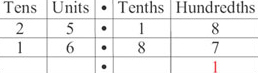In the next column (Tenths), we need to subtract 8 from 1. In order to do this, remove one unit from the next highest order of magnitude (here, units) and add this to the tenths. One unit is the same as ten tenths, so we add ten to the Tenths column: 1 tenth plus 10 tenths, gives 11 tenths in this column.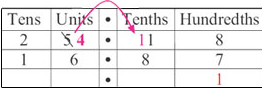Now we can subtract 8 from the resulting 11 tenths, to leave a total of 3 tenths.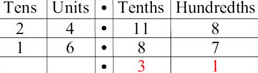In the next column (Units) we need to subtract 6 from 4, so once again we need to give this column a unit from the next highest order of magnitude (tens). 1 ten is 10 units and if we add this to the 4 units we already have, this gives 14 units in this column. Now we can subtract 6 from 14, to leave 8 units.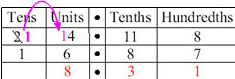Finally, in the Tens column, we're left with 1 ten to subtract from 1 ten, which leaves 0 tens.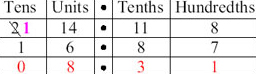So, the result of 25.18 - 16.87 = 8.31.

You can double-check you're right by adding 8.31 to 16.87 in order to confirm you get back to the original 25.18.

Now practise some subtractions for yourself with practice question 13.

Right click and open the practice questions [Tip: hold Ctrl and click a link to open it in a new tab. (Hide tip)] in a separate window, then you can switch easily between the course text and the questions.

S110_1